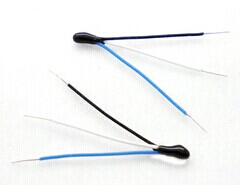2014年2月7星期五下午9:10:34NTC探头体积小便于安装且反应速度在2秒以内

R25=2.252KΩ 0.5%  B=3976K、 R25=10KΩ 0.5% B=3976K
R25=10KΩ 0.2% B=3976K、R25=6KΩ 0.5% B=3976K
R25=15KΩ 0.4% B=3600K、R25=2.252KΩ 0.1% B=3935K
R25=2.3KΩ 0.01% B=3950K、R25:100.0KΩ±0.5%,B:3976K±0.5%
R25=6.8KΩ 0.03% B=3950K、R25:200.0KΩ±0.5%,B:3976K±0.5%
R37℃=60.06KΩ±5‰  B25/50=4000±5‰
R37℃=18KΩ±3‰  B25/50=3936A±5‰
R25=10KΩ B25/50=3935